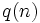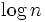# Largest prime power divisor

(diff) ← Older revision | Latest revision (diff) | Newer revision → (diff)
This article defines an arithmetic function or number-theoretic function: a function from the natural numbers to a ring (usually, the ring of integers, rational numbers, real numbers, or complex numbers).
View a complete list of arithmetic functions

## Definition

Letbe a natural number. The largest prime power divisor of, sometimes denotedand sometimes denoted, is defined as the largest prime power that divides.

## Behavior

The ID of the sequence in the Online Encyclopedia of Integer Sequences is A034699

### Lower bound

Further information: Largest prime power divisor has logarithmic lower bound

The largest prime power divisor ofis greater than. Thus, we have:.

### Asymptotic fraction

Further information: Fractional distribution of largest prime power divisor

The value ofis almost uniformly distributed in the interval.